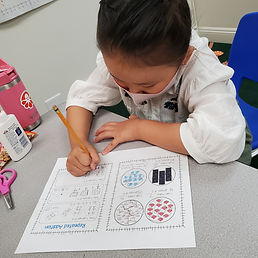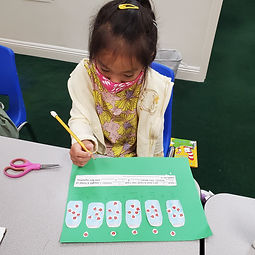## Ms. Raejean

### Target 1​

###### Lesson Type:

Continuation

Number Operation

:

Computation

Work with equal groups of numbers to gain foundations for multiplication (repeated addition).

###### 2:

Set the foundation for later exploration of multiplication (i.e. 2+2+2+2 =? How many 2s did you add? When you add 4 number 2s together, what do you get?)

###### 3:

Use addition to find the total number of objects in an array.

2nd

###### Vocabulary:

Activities:

Students continued to practice repeated addition to count a group of objects instead of counting by ones. Students broke a large group of objects into smaller groups and used repeated addition to determine how many are in the whole group. This helps to develop their foundational understanding of multiplication. We discussed how many times a number was added. Ex. How many times did you add 4? When you added 4 six times, what did you get?

Students also made their own “problem.” They put ladybugs in jars and identified the repeated addition equation, as well as the matching multiplication equation. They used repeated addition to determine how many ladybugs were in all. Ex. 5 ladybugs in 6 jars = 5 + 5 + 5 + 5 + 5 + 5. One way students solved this was by grouping the 5s to make 10. So 5 + 5 + 5 + 5 + 5 + 5 = 10 + 10 + 10 = 30.### Home Exploration

###### Guiding Questions:## Absent Students:

### Target 2

:

###### 1:

Measure and compare length in nonstandard units.

###### 2:

Understand that a non-standard unit must be placed end-to-end (with no gaps or overlap).

2nd

###### Vocabulary:

Measure, Non-Standard Units

Activities:

Measuring with non-standard units was introduced. Students developed their understanding by measuring heights with non-standard units (Ex. a pencil, the horn of a narwhal). We talked about where to start and end the measuring, and moving the tool carefully to measure without gaps and overlaps.### Home Exploration

###### Guiding Questions:### Target 3

:

###### Vocabulary:

Activities:### Home Exploration